# Spectral Enhancement

## Producer Field Guide

HGD_Product
Producer Field Guide
HGD_Portfolio_Suite
Producer

The enhancement techniques that follow require more than one band of data. They can be used to:

• compress bands of data that are similar
• extract new bands of data that are more interpretable to the eye
• apply mathematical transforms and algorithms
• display a wider variety of information in the three available color guns (R, G, B)

In this documentation, some examples are illustrated with two-dimensional graphs. However, you are not limited to two-dimensional (two-band) data. ERDAS IMAGINE programs allow an unlimited number of bands to be used. Keep in mind that processing such data sets can require a large amount of computer swap space. In practice, the principles outlined below apply to any number of bands.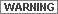Some of these enhancements can be used to prepare data for classification. However, this is a risky practice unless you are very familiar with your data and the changes that you are making to it. Anytime you alter values, you risk losing some information.

Principal Components Analysis

Principal components analysis (PCA) is often used as a method of data compression. It allows redundant data to be compacted into fewer bands—that is, the dimensionality of the data is reduced. The bands of PCA data are noncorrelated and independent, and are often more interpretable than the source data (Jensen, 1996; Faust, 1989).

The process is easily explained graphically with an example of data in two bands. Below is an example of a two-band scatterplot, which shows the relationships of data file values in two bands. The values of one band are plotted against those of the other. If both bands have normal distributions, an ellipse shape results.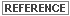Scatterplots and normal distributions are discussed in Math Topics.

Two Band Scatterplot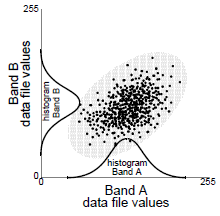Ellipse Diagram

In an n-dimensional histogram, an ellipse (2 dimensions), ellipsoid (3 dimensions), or hyperellipsoid (more than 3 dimensions) is formed if the distributions of each input band are normal or near normal. The term ellipse is used for general purposes here.

To perform PCA, the axes of the spectral space are rotated, changing the coordinates of each pixel in spectral space, as well as the data file values. The new axes are parallel to the axes of the ellipse.

First Principal Component

The length and direction of the widest transect of the ellipse are calculated using matrix algebra in a process explained below. The transect, which corresponds to the major (longest) axis of the ellipse, is called the first principal component of the data. The direction of the first principal component is the first eigenvector, and its length is the first eigenvalue (Taylor, 1977).

A new axis of the spectral space is defined by this first principal component. The points in the scatterplot are now given new coordinates, which correspond to this new axis. Since, in spectral space, the coordinates of the points are the data file values, new data file values are derived from this process. These values are stored in the first principal component band of a new data file.

First Principal Component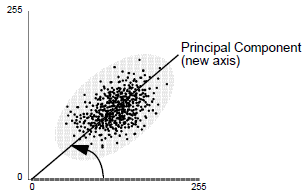The first principal component shows the direction and length of the widest transect of the ellipse. Therefore, as an axis in spectral space, it measures the highest variation within the data. In the figure below it is easy to see that the first eigenvalue is always greater than the ranges of the input bands, just as the hypotenuse of a right triangle must always be longer than the legs.

Range of First Principal Component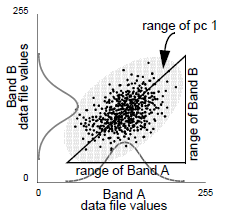Successive Principal Components

The second principal component is the widest transect of the ellipse that is orthogonal (perpendicular) to the first principal component. As such, the second principal component describes the largest amount of variance in the data that is not already described by the first principal component (Taylor, 1977). In a two-dimensional analysis, the second principal component corresponds to the minor axis of the ellipse.

Second Principal Component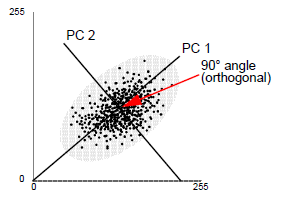In n dimensions, there are n principal components. Each successive principal component:

• is the widest transect of the ellipse that is orthogonal to the previous components in the n-dimensional space of the scatterplot (Faust, 1989), and
• accounts for a decreasing amount of the variation in the data which is not already accounted for by previous principal components (Taylor, 1977).

Although there are n output bands in a PCA, the first few bands account for a high proportion of the variance in the data—in some cases, almost 100%. Therefore, PCA is useful for compressing data into fewer bands.

In other applications, useful information can be gathered from the principal component bands with the least variance. These bands can show subtle details in the image that were obscured by higher contrast in the original image. These bands may also show regular noise in the data (for example, the striping in old MSS data) (Faust, 1989).

Computing Principal Components

To compute a principal components transformation, a linear transformation is performed on the data. This means that the coordinates of each pixel in spectral space (the original data file values) are recomputed using a linear equation. The result of the transformation is that the axes in n-dimensional spectral space are shifted and rotated to be relative to the axes of the ellipse.

To perform the linear transformation, the eigenvectors and eigenvalues of the n principal components must be mathematically derived from the covariance matrix, as shown in the following equation: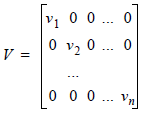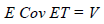Where:

Cov = covariance matrix

E = matrix of eigenvectors

T = transposition function

V = a diagonal matrix of eigenvalues, in which all nondiagonal elements are zeros

V is computed so that its nonzero elements are ordered from greatest to least, so that

Source: Faust, 1989A full explanation of this computation can be found in Gonzalez and Wintz, 1977.

The matrix V is the covariance matrix of the output principal component file. The zeros represent the covariance between bands (there is none), and the eigenvalues are the variance values for each band. Because the eigenvalues are ordered from v1 to vn, the first eigenvalue is the largest and represents the most variance in the data.

Each column of the resulting eigenvector matrix, E, describes a unit-length vector in spectral space, which shows the direction of the principal component (the ellipse axis). The numbers are used as coefficients in the following equation, to transform the original data file values into the principal component values.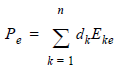Where:

e = number of the principal component (first, second)

Pe = output principal component value for principal component number e

k = a particular input band

n = total number of bands

dk = an input data file value in band k

Eke = eigenvector matrix element at row k, column e

Source: Modified from Gonzalez and Wintz, 1977

Decorrelation Stretch

The purpose of a contrast stretch is to:

• alter the distribution of the image DN values within the 0 - 255 range of the display device, and
• utilize the full range of values in a linear fashion.

The decorrelation stretch stretches the principal components of an image, not to the original image.

A principal components transform converts a multiband image into a set of mutually orthogonal images portraying inter-band variance. Depending on the DN ranges and the variance of the individual input bands, these new images (PCs) occupy only a portion of the possible 0 - 255 data range.

Each PC is separately stretched to fully utilize the data range. The new stretched PC composite image is then retransformed to the original data areas.

Either the original PCs or the stretched PCs may be saved as a permanent image file for viewing after the stretch.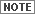Storage of PCs as floating point, single precision is probably appropriate in this case.

Tasseled Cap

The different bands in a multispectral image can be visualized as defining an N-dimensional space where N is the number of bands. Each pixel, positioned according to its DN value in each band, lies within the N-dimensional space. This pixel distribution is determined by the absorption/reflection spectra of the imaged material. This clustering of the pixels is termed the data structure (Crist and Kauth, 1986).See Raster Data for more information on absorption/reflection spectra. See the discussion on Principal Components Analysis earlier in this topic.

The data structure can be considered a multidimensional hyperellipsoid. The principal axes of this data structure are not necessarily aligned with the axes of the data space (defined as the bands of the input image). They are more directly related to the absorption spectra. For viewing purposes, it is advantageous to rotate the N-dimensional space such that one or two of the data structure axes are aligned with the Viewer X and Y axes. In particular, you could view the axes that are largest for the data structure produced by the absorption peaks of special interest for the application.

For example, a geologist and a botanist are interested in different absorption features. They would want to view different data structures and therefore, different data structure axes. Both would benefit from viewing the data in a way that would maximize visibility of the data structure of interest.

Tasseled Cap transformation provides useful information for agricultural applications because it allows the separation of barren (bright) soils from vegetated and wet soils. Research has produced three data structure axes that define the vegetation information content (Crist et al, 1986, Crist and Kauth, 1986):

• Brightness—a weighted sum of all bands, defined in the direction of the principal variation in soil reflectance.
• Greenness—orthogonal to brightness, a contrast between the near-infrared and visible bands. Strongly related to the amount of green vegetation in the scene.
• Wetness—relates to canopy and soil moisture (Lillesand and Kiefer, 1987).

A simple calculation (linear combination) then rotates the data space to present any of these axes to you.

The resulting RGB color composite image shows information about the various states of cultivated fields in the image.

• Bright areas (sand, barren land) appear in red
• Crops under cultivation appear in green or cyan
• Fallow fields appear brownish
• Water areas appear bright blue

This is a traditional way of displaying the three Tasseled Cap images as an RGB color composite, and is especially useful for assessing the cultivated states of agricultural areas.

These rotations are sensor-dependent, but once defined for a particular sensor (say Landsat 4 TM), the same rotation works for any scene taken by that sensor. The increased dimensionality (number of bands) of TM vs. MSS allowed Crist et al (Crist et al, 1986) to define three additional axes, termed Haze, Fifth, and Sixth. Lavreau (Lavreau, 1991) has used this haze parameter to devise an algorithm to dehaze Landsat imagery.

The Landsat TM formulas multiply Landsat TM bands 1-5 and 7 by weighted coefficients. For TM4, the calculations are:

Brightness = .3037 (TM1) + .2793 (TM2) + .4743 (TM3) + .5585 (TM4) + .5082 (TM5) + .1863 (TM7)

Greenness = .2848 (TM1) - .2435 (TM2) - .5436 (TM3) + .7243 (TM4) + .0840 (TM5) - .1800 (TM7)

Wetness = .1509 (TM1) + .1973 (TM2) + .3279 (TM3) + .3406 (TM4) - .7112 (TM5) - .4572 (TM7)

Haze = .8832 (TM1) - .0819 (TM2) - .4580 (TM3) - .0032 (TM4) - .0563 (TM5) + .0130 (TM7)

Source: Modified from Crist et al, 1986, Jensen, 1996

RGB to IHS

The color monitors used for image display on image processing systems have three color filters or guns. These correspond to red, green, and blue (R,G,B), the additive primary colors. When displaying three bands of a multiband data set, the viewed image is said to be in R,G,B space.

However, it is possible to define an alternate color space that uses intensity (I), hue (H), and saturation (S) as the three positioned parameters (in lieu of R,G, and B). This system is advantageous in that it presents colors more nearly as perceived by the human eye.

• Intensity is the overall brightness of the scene (like PC-1) and varies from 0 (black) to 1 (white).
• Saturation represents the purity of color and also varies linearly from 0 to 1.
• Hue is representative of the color or dominant wavelength of the pixel. It varies from 0 at the red midpoint through green and blue back to the red midpoint at 360. It is a circular dimension. In the following figure, 0 to 255 is the selected range; it could be defined as any data range. However, hue must vary from 0 to 360 to define the entire sphere (Buchanan, 1979).

Intensity, Hue, and Saturation Color Coordinate System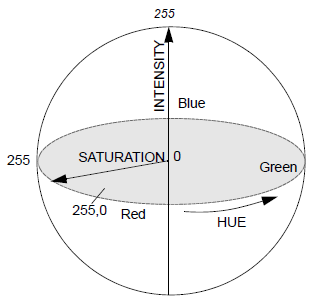Source: Buchanan, 1979

The algorithm used in Spectral Enhancement RGB to IHS transform is (Conrac Corporation, 1980):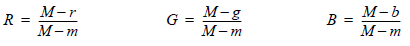Where:

R,G,B are each in the range of 0 to 1.0.

r, g, b are each in the range of 0 to 1.0.

M = largest value, r, g, or b

m = least value, r, g, or bAt least one of the R, G, or B values is 0, corresponding to the color with the largest value, and at least one of the R, G, or B values is 1, corresponding to the color with the least value.

The equation for calculating intensity in the range of 0 to 1.0 is: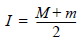The equations for calculating saturation in the range of 0 to 1.0 are: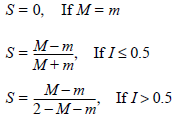The equations for calculating hue in the range of 0 to 360 are:

If M = m, H = 0

If r = M, H = 60(2 + b - g)

If g = M, H = 60(4 + r - b)

If b = M, H = 60(6 + g - r)

Where:

r,g,b are each in the range of 0 to 1.0.

M = largest value, r, g, or b

m = least value, r, g, or b

If the resulting hue is greater than 360, 360 is subtracted so that the result is between 0 and 360.

IHS to RGB

The family of IHS to RGB is intended as a complement to the standard RGB to IHS transform.

In the IHS to RGB algorithm, a min-max stretch is applied to either intensity (I), saturation (S), or both, so that they more fully utilize the 0 to 1 value range. The values for hue (H), a circular dimension, are 0 to 360. However, depending on the dynamic range of the DN values of the input image, it is possible that I or S or both occupy only a part of the 0 to 1 range. In this model, a min-max stretch is applied to either I, S, or both, so that they more fully utilize the 0 to 1 value range. After stretching, the full IHS image is retransformed back to the original RGB space. As the parameter Hue is not modified, it largely defines what we perceive as color, and the resultant image looks very much like the input image.

It is not essential that the input parameters (IHS) to this transform be derived from an RGB to IHS transform. You could define I or S as other parameters, set Hue at 0 to 360, and then transform to RGB space. This is a method of color coding other data sets.

In another approach (Daily, 1983), H and I are replaced by low- and high-frequency radar imagery. You can also replace I with radar intensity before the IHS to RGB transform (Croft (Holcomb), 1993). Chavez evaluates the use of the IHS to RGB transform to resolution merge Landsat TM with SPOT panchromatic imagery (Chavez et al, 1991).Use the Spatial Modeler for this analysis.See the previous section on RGB to IHS transform for more information.

The algorithm used by ERDAS IMAGINE for the IHS to RGB function is (Conrac Corporation, 1980):

Given: H in the range of 0 to 360; I and S in the range of 0 to 1.0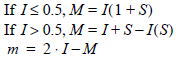The equations for calculating R in the range of 0 to 1.0 are: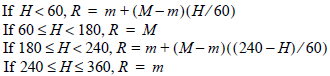The equations for calculating G in the range of 0 to 1.0 are: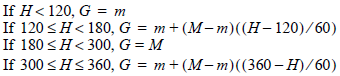Equations for calculating B in the range of 0 to 1.0: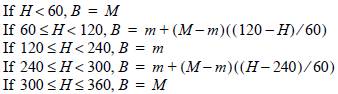Indices

Indices are used to create output images by mathematically combining the DN values of different bands. These may be simplistic:

(Band X - Band Y)

or more complex: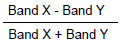In many instances, these indices are ratios of band DN values: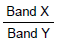These ratio images are derived from the absorption/reflection spectra of the material of interest. The absorption is based on the molecular bonds in the (surface) material. Thus, the ratio often gives information on the chemical composition of the target.See Raster Data for more information on the absorption and reflection spectra.

The technique of ratioing bands involves dividing the spectral response value of a pixel in one image with the spectral value of the corresponding pixel in another image. This is done in order to suppress similarities between bands. This is useful for eliminating albedo effects and shadows.

Applications

• Indices are used extensively in mineral exploration and vegetation analysis to bring out small differences between various rock types and vegetation classes. In many cases, judiciously chosen indices can highlight and enhance differences that cannot be observed in the display of the original color bands.
• Indices can also be used to minimize shadow effects in satellite and aircraft multispectral images. Black and white images of individual indices or a color combination of three ratios may be generated.
• Certain combinations of TM ratios are routinely used by geologists for interpretation of Landsat imagery for mineral type. For example: Red 5/7, Green 5/4, Blue 3/1.

Integer Scaling Considerations

The nature of band ratioing is that every pixel that has the same spectral response between input bands will have a value of 1 in the output image; deviations from 1 indicating progressively different initial spectral values. Areas of greatest change are found in the tails of the resultant histogram. Production of a change image will involve thresholding the image histogram to suppress those areas where little or no change has occurred.

The output images obtained by applying indices are generally created in floating point to preserve all numerical precision. If there are two bands, A and B, then:

ratio = A/B

If A>>B (much greater than), then a normal integer scaling would be sufficient. If A>B and A is never much greater than B, scaling might be a problem in that the data range might only go from 1 to 2 or from 1 to 3. In this case, integer scaling would give very little contrast.

For cases in which A<B or A<<B, integer scaling would always truncate to 0. All fractional data would be lost. A multiplication constant factor would also not be very effective in seeing the data contrast between 0 and 1, which may very well be a substantial part of the data image. One approach to handling the entire ratio range is to actually process the function:

ratio = atan(A/B)

This would give a better representation for A/B < 1 as well as for A/B > 1.

Index Examples

The following are examples of indices that are in Classification group in ERDAS IMAGINE:

• IR/R (infrared / red)
• SQRT (IR/R)
• Vegetation Index = IR - R
• Normalized Difference Vegetation Index: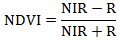• Transformed NDVI: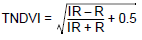• Iron Oxide = TM 3/1
• Clay Minerals = TM 5/7
• Ferrous Minerals = TM 5/4
• Mineral Composite = TM 5/7, 5/4, 3/1
• Hydrothermal Composite = TM 5/7, 3/1, 4/3

Source: Modified from Sabins, 1987; Jensen, 1996; Tucker, 1979

The following table shows the infrared (IR) and red (R) band for some common sensors (Tucker, 1979, Jensen, 1996):

 Sensor IR Band R Band Landsat MSS 7 5 SPOT XS 3 2 Landsat TM 4 3 NOAA AVHRR 2 1

Vegetation Indices

An assumption of vegetation indices is the idea that all bare soil in an image will form a line in spectral space. Nearly all of the commonly used vegetation indices are only concerned with red-near-infrared space, so a red-near-infrared line for bare soil is assumed. This line is considered to be the line of zero vegetation.

At this point, there are two divergent lines of thinking about the orientation of lines of equal vegetation (isovegetation lines):

1. All isovegetation lines converge at a single point. The indices that use this assumption are the ratio-based indices, which measure the slope of the line between the point of convergence and the red-NIR point of the pixel. Some examples are: NDVI (Normalized Difference Vegetation Index) and RVI (Ratio Vegetation Index -- also known as Band Ratios).
2. All isovegetation lines remain parallel to soil line. These indices are typically called perpendicular indices and they measure the perpendicular distance from the soil line to the red-NIR point of the pixel. Examples are: PVI (Perpendicular Vegetation Index) and DVI (Difference Vegetation Index).

Vegetation formulas should be used only with data containing three or more bands.

Image Algebra

Image algebra is a general term used to describe operations that combine the pixels of two or more raster layers in mathematical combinations. For example, the calculation:

(infrared band) - (red band)

DNir - DNred

yields a simple, yet very useful, measure of the presence of vegetation. At the other extreme is Tasseled Cap calculation, which uses a more complicated mathematical combination of as many as six bands to define vegetation.

Band ratios, such as: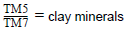are also commonly used. These are derived from the absorption spectra of the material of interest. The numerator is a baseline of background absorption and the denominator is an absorption peak.See Raster Data for more information on absorption and reflection spectra.

NDVI is a combination of addition, subtraction, and division: